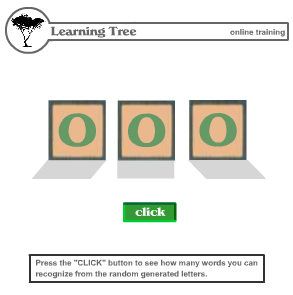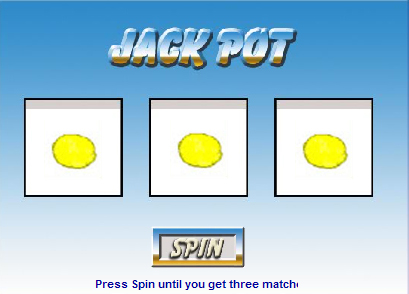cnjmug
 level - Intermediate In Actionscript you can emulate a simply random math function such as rolling a dice. When you roll a dice you have a one in six chance of getting a number one through six. You can construct a simple flash game to illustrate this function. The first thing we need to do is create a movie clip that has six frames displaying each side of the dice. Then we need to create a button called "random". If the project is done correctly the dice should reveal a number when the button is pressed. Below is the code for this simple game. (I am using ActionScript 3 here. The basic principal of this code is identical in ActionScript 2.) random.mc.addeventListener(MouseEvent.Click, onClick); function onClick(event:MouseEvent):void { dice_mc.gotoAndStop(Math.ceil(Math.random() * 6)); } Dice (dice_mc) is our movie clip with the six sides of the dice represented in its frames. The Math.random() * 6 code randomly selects one of the six frames when the button random_mc is pressed. The gotoAndStop function simply stops the frame that has been randomly selected. What if you wanted to randomly select letters of the alphabet. The code would be virtually the same as the one we used for the dice program. The main change would be changing the 6 to 26 in the Math.random code since there are 26 letters in the alphabet. Of course you would have to list all the letters of the alphabet in the frames of the movie clip (letters_mc). An example of the main code is below. letters_mc.gotoAndStop(Math.ceil(Math.random() * 26)); All that has been changed here is the dice movie clip is now called letters and the random number has been changed from 6 to 26. To make this project more interesting we could create a flash program that randomly spells a three letter word. We now would create three movie clips randomly generating letters. You would keep on pressing the random button until you spelled a word. Here is a sample of that code. random.mc.addeventListener(MouseEvent.Click, onClick); function onClick(event:MouseEvent):void { letters1_mc.gotoAndStop(Math.ceil(Math.random() * 26)); letters2_mc.gotoAndStop(Math.ceil(Math.random() * 26)); letters3_mc.gotoAndStop(Math.ceil(Math.random() * 26)); } A sample of this project is on the top of the next column.Generating a random word. Click here to see sample We could also use similar code to create a slot machine game. Like the word generating code we would have three movie clips generating frame objects. This time the frame objects are 5 fruits and one number 7 instead of the 26 letters of the alphabet. The ActionScript code would look like this. spin.mc.addeventListener(MouseEvent.Click, onClick); function onClick(event:MouseEvent):void { matches1_mc.gotoAndStop(Math.ceil(Math.random() * 6)); matches2_mc.gotoAndStop(Math.ceil(Math.random() * 6)); matches3_mc.gotoAndStop(Math.ceil(Math.random() * 6)); } Below is the game based on the above code.Slot Machine Game Click here to see sample
© 2008 JAM - Jersey Adobe Multimedia. Created April 15, 2008